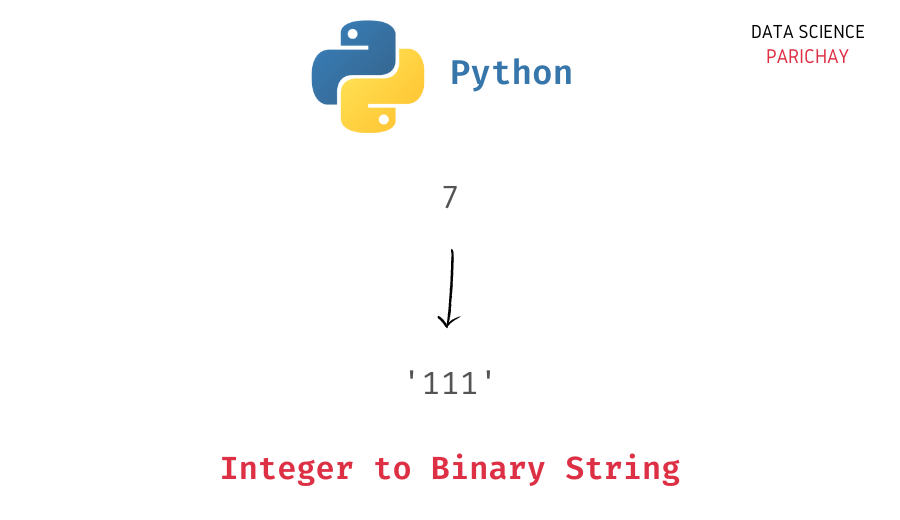# Python – Convert Integer to Binary String

In this tutorial, we will look at how to convert an integer to a binary string in Python with the help of examples.

## How to convert int to binary in Python?

You can use the Python built-in `bin()` function to convert an integer to a binary string. Pass the integer as an argument. The following is the syntax:

```# int i to binary string
bin(i)```

It returns the binary representation of the integer in a string format.

Let’s look at an example.

```# integer
num = 7
# integer to binary
num_bin = bin(num)
# display binary string and its type
print(num_bin)
print(type(num_bin))```

Output:

```0b111
<class 'str'>```

Here, we convert the number `7` to a binary string. You can see that we get `'0b111'` as the output. Note that the binary representation `111` has a prefix `0b`.

You can use a string slice operation to remove this prefix.

```# integer to binary
num_bin = bin(num)[2:]
print(num_bin)```

Output:

📚 Data Science Programs By Skill Level

Introductory

Intermediate ⭐⭐⭐

🔎 Find Data Science Programs 👨‍💻 111,889 already enrolled

Disclaimer: Data Science Parichay is reader supported. When you purchase a course through a link on this site, we may earn a small commission at no additional cost to you. Earned commissions help support this website and its team of writers.

`111`

We get the binary string for `7` without the `0b` prefix.

### Int to binary using `format()`

Alternatively, you can use the Python `format()` function. It returns the formatted representation of the passed value. Pass the value and the format specification as arguments to the function. To convert a value to binary, use `'b'` as the format specification.

Let’s look at an example.

```# integer
num = 7
# integer to binary
num_bin = format(num, 'b')
# display binary string and its type
print(num_bin)
print(type(num_bin))```

Output:

```111
<class 'str'>```

Here we again convert `7` to a binary string. You can see that we get `'111'` as the output. Notice that unlike the `bin()` function above, this function does not add any prefix to the binary string.

You might also be interested in –

•